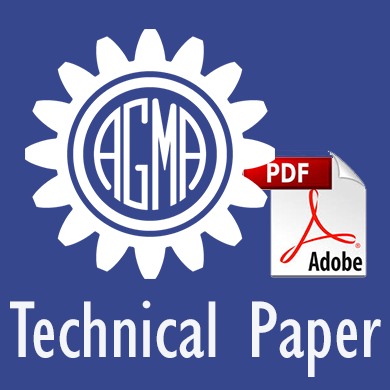CustomProperty
Use of Duty Cycles or Measured Torque-Time Data with AGMA Ratings

## 21FTM07Use of Duty Cycles or Measured Torque-Time Data with AGMA Ratings

Nowadays continuous torque measurement on gearboxes is increasingly popular, not only on very sensitive installations but also on many industrial gearboxes and wind turbines. When the transmitted load is not uniform, consideration should be given not only to the peak load and its anticipated number of cycles, but also to intermediate loads and their numbers of cycles. This type of load is considered a duty cycle and may be represented by a load spectrum. In such cases, the cumulative fatigue effect of the duty cycle is considered in rating the gear set. A method of calculating the effect of the loads under these conditions, such as Miner’s Rule, is not explained in AGMA rating methods (as AGMA 2001, 2101, 2003) but a reference is given to ISO/TR 10495 (nowadays replaced by ISO 6336-6:2019).

In this paper the application of torque spectra in AGMA rating methods is described. Furthermore, a procedure to convert measured (or calculated by numerical simulation) torque data into a torque spectrum according to the definition in ISO 6336-6 is described. This task is simple if the torque is always positive, but quite complicated when also negative torque sequences happen.

The torque spectrum must represent the load on each single tooth, therefore in a first step the continuous torque course has to be segmented in individual torque peaks applied on one tooth. If only positive torque occurs (no load reversal), the "Simple Count" method can be used. The method counts how often a torque value happens to be in a certain torque range. A tooth is always loaded by a torque value starting at zero to a peak, hence it is subjected to pulsating stress (stress ratio R=0). Tooth bending stress calculation according to AGMA is assuming pulsating stress, so the result of the “Simple Count” method can directly be used for the tooth bending strength verification.

For complex loads, where the torque has both positive and negative signs, the “Rainflow method” (ISO 12110-2) should be applied. 'Rainflow Counting' is a method to determine the number of fatigue cycles present in a load-time history. The method is used in the analysis of fatigue data in order to reduce a spectrum of varying stress into an equivalent set of simple stress reversals. So, a tooth is loaded by cycles with a high and a low torque (respectively stress), having a variable stress ratio R ≠ 0. To comply with the rules of AGMA ratings, a reverse loading factor must be used to modify the admitted sat values for tooth bending. In ISO, this factor is the mean stress influence factor YM which must be defined for every bin of the torque spectrum.

In the final part of this paper a practical application of the above method is presented.

Author: Ulrich Kissling

ISBN: 978-1-64353-101-4

Discounted member price: 65.00
85.00
You could save: 23.5%